# A Precision Advertising Strategy Based on Deep Reinforcement Learning

A Precision Advertising Strategy Based on Deep Reinforcement Learning
Ulanqab Vocational College, Ulanqab 012000, China

Corresponding Author Email:
nmglhq1984@163.com
Page:
397-403
|
DOI:
https://doi.org/10.18280/isi.250316
2 February 2020
|
Accepted:
3 May 2020
|
Published:
30 June 2020
| Citation

OPEN ACCESS

Abstract:

Based on big data analysis, precision advertising fully meets the needs of users, and boasts a high application value. From the perspective of deep reinforcement learning (DRL), this paper attempt to develop a precision advertising strategy, capable of extracting effective features from massive advertising data and predicting advertising precision accurately and efficiently. Firstly, the advertising data were preprocessed, and organized into an advertising data sequence, in which the data are intercorrelated. In addition, the feature construction process was detailed. After that, a prediction model of advertising precision was developed in three steps, based on the Q-learning algorithm. The proposed strategy was found to be effective and accurate through experiments. The research results provide a reference for applying Q-learning to precision prediction in other fields.

Keywords:

deep reinforcement learning (DRL), precision advertising, Q-learning, feature extraction

1. Introduction

With the advancement of social technology and informatization, the advertising industry is vigorously seeking innovation, optimization, and upgrading. Advertising companies have attached greater importance to the reach percentage and click-through rate (CTR) and attempted to establish personalized advertising systems, in a bid to enhance the communication value and economic benefits of ads [1-4]. To realize accurate, timely, and effective advertising, a feasible and viable strategy is to adopt big data mining technology, and implement personalized analysis on user behaviors and advertising data, and thus identify potential users [5-9].

In general, big data analysis has not been widely applied to implement precision advertising, but to construct user portraits [20-22]. Xiao et al.  analyzed user portrayal model of JD Shufang, which relies on the 4A (Aware, Appeal, Ask, and Advocate) model to analyze user information in real time. Lee et al.  developed a content-based algorithm and a graph-based algorithm, both of which can portrait users based on their consumption features. With the aid of Word2vec, Zeng et al.  conducted multi-dimensional analysis on the keywords searched by users, and then performed customized analysis on user portraits using visual charts.

To sum up, there is little report that systematically discusses the feature extraction and selection of advertising data. Moreover, the relevant prediction models are inefficient and inaccurate. To overcome these defects, this paper tries to develop a precision advertising strategy based on deep reinforcement learning (DRL). Firstly, the erroneous, redundant, and missing items in advertising data were preprocessed. Then, the correlations between data in the advertising data sequence were sorted out, and the feature construction process was detailed. After that, a prediction model of advertising precision was developed in three steps, based on the Q-learning algorithm oriented at precision advertising. The proposed precision advertising strategy was proved effective and accurate through experiments.

The original dataset was constructed by summarizing, classifying, and integrating the following data in advertising logs on Apache Flume: historical data on user behaviors, and basic advertising data. Among them, the historical data on user behaviors mainly the search keywords, the viewing completion, the clicking time, and the consumption time. The basic advertising data mainly cover creative keywords, total clicks, CTR, consumption rate, ranking, advertising time, and advertising frequency. Among them, the ranking can be calculated by:

$R_{\mathrm{avg}}=\sum_{i=1}^{N} R_{i} / N$        (1)

where, N is the total clicks; Ri is the ranking of the i-th ad.

## 1.pngFigure 1. The procedure of feature construction

Next, the original dataset was preprocessed into an effectively data sequence for the training of the DRL network. Specifically, the original dataset was cleaned to remove erroneous and redundant items. Besides, the missing items, no more than 1/5 of the total amount of data, were imputed multiple times through logistic regression.

In the original dataset, many items were missing in the categories of viewing completion, advertising time, advertising frequency, and consumption time. The imputation results of these items are displayed in Tables 1 and 2.

After setting up the advertising data sequence, the correlations of the data in the sequence were sorted out. From the angle of users, the viewing completion of a user on an ad is positively correlated with the probability that he/she click on the same ad at the next moment. From the angle of ad, the relationship between advertising time and the CTR exhibited a statistical regularity.

Then, the ads were divided into multiple classes by the creative keywords. It was learned that the CTRs of ads in the same class fluctuated slightly between adjacent periods. From the perspective of ad-user correlation, the fitness between creative keywords and search keywords is positively correlated with the probability that users click on the ad.

The above data correlations were relied on to construct the DRL model and design the advertising strategy, aiming to optimize the effect of precision advertising.

The next step is to extract the features of advertising data effectively, and balance the data in different classes. Hence, the features of advertising data were constructed and then optimized. The procedure of feature construction is illustrated in Figure 1. Note that redundant data removal is to delete the features that can be characterized by other features, aiming to shorten the training of the DRL model. Here, only 4 features of the historical data on user behaviors and 7 features on basic advertising data are retained for clustering and optimization.

Feature optimization aims to highlight and optimize the salient features. Since the DRL model needs to consider the fitness between creative and search keywords, the corresponding tags were attached to the characteristic keywords. Based on the keywords, the feature data with large magnitudes (e.g. high ranking) were normalized by interval scaling into [0, 1].

After the above preprocessing, the feature information was obtained as shown in Table 3.

Table 1. Imputation methods and categories of missing items

 Imputation method Category Fully conditional iteration Click time, consumption time, advertising time, viewing completion, advertising frequency Imputed Viewing completion, advertising frequency Not imputed (too many missing items) / Not imputed (too many missing items) Clicks, click rate, consumption rate, ranking Imputation from sequence Search keywords, creative keywords

Table 2. Imputation information

 Category Type of model Missing items Imputed items Viewing completion Linear regression 402 402 Advertising time Linear regression 113 113 Advertising frequency Linear regression 102 102 Consumption time Linear regression 658 658

Table 3. The feature information of advertising data

 Category Name Type User behavior features Search keywords Text features Viewing completion Digital features Click time Time features Consumption time Time features Ad features Creative keywords Text features Clicks Digital features CTR Digital features Consumption rate Digital features Ranking Digital features Advertising time Time features Advertising frequency Digital features
3. Q-Learning Algorithm

The DRL usually involves two entities: the agent and the environment. Let st be the state perceived by the agent in the environment, at be the action taken by the agent, and rt be the reward obtained by the agent for taking the action at under state st, where t is the time period. Then, the interaction between the agent and the environment can be viewed as a Markov decision process (as shown in Figure 2 below):

The environment provides the agent with the state st, under which the agent takes the action at on the environment; after receiving the reward rt from the environment, the agent enters into a new state st+1; then, the agent will take another action under the new agent, receive the corresponding reward, and enter a newer state…

In the Markov decision process, each action at leads to a unique reward rt, which is dependent on the corresponding time period t.

## 2.png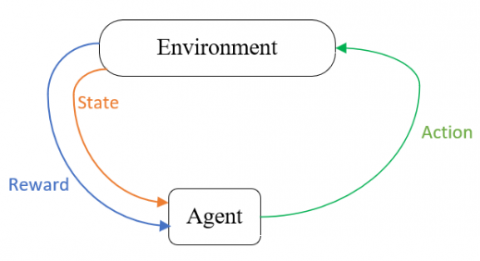Figure 2. The Markov decision process

The biggest difference between Q-learning algorithm and DRL is that the algorithm is not based on the entity of environment, but measures the state value with a Q function. The algorithm allows the agent to choose between real-time reward or delayed reward. Here, the feature information is imported to the following formula for iterative update:

\begin{align} & {{Q}_{t+1}}\left( {{s}_{t}},{{a}_{t}} \right)={{Q}_{t}}\left( {{s}_{t}},{{a}_{t}} \right)+\alpha \left[ {{Q}_{\text{target}}}\left( {{s}_{t}},{{a}_{t}} \right)-{{Q}_{t}}\left( {{s}_{t}},{{a}_{t}} \right) \right] \\ & {{Q}_{\text{target}}}\left( {{s}_{t}},{{a}_{t}} \right)={{r}_{t+1}}+\gamma \underset{{{a}'}}{\mathop{\max }}\,{{Q}_{t+1}}\left( {{s}_{t+1}},{a}' \right) \\ \end{align}          (2)

where, Qtarget(st, at) is the target value of Q-learning. It can be seen from formula (2) that, under a given state st, the target value decays by γ times after action at is taken; then, the agent and the environment will interact by a learning strategy α to obtain the cumulative expectation, that is, to approximate the optimal Q function through accumulation.

Because the features of advertising data are high-dimensional, the traditional Q-learning algorithm cannot deal with the strong correlations between the user features and ad features. Therefore, Q-learning algorithm was fused into the convolutional neural network (CNN) into a deep Q network (DQN). In the DQN, the value function Q can be approximately expressed as:

$Q_{t+1}^{*}\left(s_{t}, a_{t}, \omega\right) \approx Q_{t+1}\left(s_{t}, a_{t}\right)$          (3)

## 3.png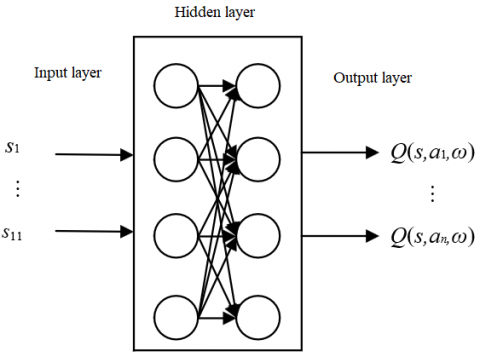Figure 3. The basic architecture of the DQN

As shown in Figure 3, the DQN predicts the Q value by neural networks, rather than record the value in a Q table. The neural networks are updated constantly to optimize the action path. There are two neural networks in the DQN, namely, target-net and eval-net. The former acquires the value of Q-target, and the latter acquires the value of Q-evaluation.

For the scenario of precision advertising, the input state is denoted as st, representing 11 advertising data features in a time period t. Then, the state of advertising effect is denoted as st=(s1, s2, …, s11). The output of the DQN is a value Q*t+1(st, aj, ω), reflecting the value function Q of action aj under state st.

The neural networks were trained by the loss function:

$L=\left(r_{t+1}+\gamma \max _{a^{\prime}} Q_{\text {target }}\left(s_{t+1}, a^{\prime}, \omega^{\prime}\right)-Q_{t}\left(s_{t}, a_{t}, \omega\right)\right)^{2}$           (4)

where, Qt(st, aj, ω) is the network output in time period t; Qtarget(st+1, a¢, ω¢) is the output value of Q-target. Network parameter ω was updated interactively, and ω¢ was obtained by delayed update:

$\omega_{t+1}=\omega_{t}+\alpha\left[\begin{array}{c}r_{t+1}+\gamma \max _{a^{\prime}} Q_{\mathrm{target}}\left(s_{t+1}, a^{\prime}, \omega^{\prime}\right) \\ -Q_{t}\left(s_{t}, a_{t}, \omega\right)\end{array}\right] \nabla Q_{t}\left(s_{t}, a_{t}, \omega\right)$                    (5)

4. DRL-Based Prediction Model for Advertising Precision

To realize precision advertising, a DRL-based prediction model was established in three steps to forecast the advertising precision.

Step 1. Setting up the state space and action space

In the model, the information received by the agent from the environment generally describes the basic states of users and ads, namely, viewing completion, real-time CTR, and tags of search keywords. Let Wk(t) be the viewing completion of user k in time period t; Ph(t) be the CTR of ad h in time period t; Fk(t) be the fitness between the tags of the search keywords of user k and the tags of the creative keywords of all ads in time period t. Then, the state space for the prediction of advertising precision can be defined as:

$S=\left[W_{k}(t), P_{h}(t), F_{k}(t)\right]$        (6)

where, the Ph(t) value can be calculated by:

$P_{h}(t)=\frac{\text {Total effective clicks in time period } t}{\text {Total number of ads served in time period t}} \times 100 \%$                            (7)

After observing the state of the environment, the agent needs to select an action from the action space A based on its own decision set. In the prediction model for advertising precision, the user action is clicking or consumption in time period t, and the ad action is serving in time period t. Hence, the action space of advertising can be defined as:

$A=\left[C_{k}(t), B_{k}(t), T_{h}(t)\right]$           (8)

where, Ck(t) is the clicking of user k in time period t; Bk(t) is the consumption of user k in time period t; Th(t) is the serving of ad h in time period t. To facilitate the processing of continuous actions, the action space was discretized into the granularity of μ:

${{A}_{\text{dis}}}=\left[ {{C}_{k}}\left( t \right),{{B}_{k}}\left( t \right),{{T}_{h}}\left( t \right)|\mu \right]$           (9)

Step 2. Establishing state-action relationship by the DQN

Firstly, the Q table was set up based on the state space and action space, and the initial fitness between states and actions were recorded. Besides the advertising time, the clicking and consumption of users are related to multiple attributes (e.g. market coverage and price) of the advertised product. Therefore, the willingness of a user to take an action (hereinafter referred to as action willingness) can be characterized by:

$\sigma \left( v,r,u \right)=v+\alpha r+\beta u-\gamma {{u}^{2}}$           (10)

where, v is the expected price of the advertised product; r is the market coverage of the product; u is the effectiveness of feature extraction from advertising data. If the r is large, the influence of advertising precision Aa over action willingness Ub is mainly manifested on the expected price v and actual price p of the product:

$\frac{\partial \sigma (v,r,u)}{\partial u}=\frac{\partial v}{\partial u}=\frac{\partial p}{\partial u}$            (11)

## 4.pngFigure 4. The relationship between action willingness and advertising precision

The relationship between action willingness and advertising precision is shaped like an inverted U (as shown in Figure 4 above).

If the r is small, the users have limited knowledge and access to the product. Then, the action willingness could be greatly enhanced by increasing the advertising precision. Setting the ratio of user utility to marginal price increment σu of product to 1/3, we have:

$\frac{\partial p}{\partial u}=\frac{3{{\sigma }_{u}}(v,r,u)}{4}$         (12)

If the change of action willingness induced by advertising precision is treated as the effect of precision advertising, it can be assumed that the advertising company and users share the results of precision advertising at a small r value.

During the iteration, all variables must obey the constraints on the effect of precision advertising on action willingness. In addition, the current round of learning should terminate automatically and a new round of learning should start, whenever the fitness between action willingness and the current state becomes too low. Through the iteration, the final Q table can characterize the matching between state space and action space.

Step 3. Weighing the indices

Let A1, A2, …, An be the random variables corresponding to actions a1, a2, …, an, respectively. Then, λ1, λ2, …, λn that maximize the independence of each action satisfy:

$\left\{ \begin{matrix} MaxVar({{\lambda }_{1}}{{R}_{1}},{{\lambda }_{2}}{{R}_{2}},\cdots ,{{\lambda }_{n}}{{R}_{n}}) \\ \lambda _{1}^{2}+\lambda _{2}^{2}+\cdots +\lambda _{n}^{2}=1 \\ \end{matrix} \right.$                   (13)

Then, λ1, λ2, …, λn were taken as the weights of the actions in the final Q table. Based on these weights and the previously mentioned constraints, each state and its corresponding action could be quantified to reflect the precision of advertising.

5. Experiments and Results Analysis

To verify its effectiveness, the proposed model was applied to predict the reach percentages of four kinds of ads served in different time periods. According to the prediction results (Figure 5), the reach percentages of the different kinds of ads were very close in the same time period, despite an extremely small fluctuation. The results show that our model can adapt to the advertising time to a certain extent, and output predictions within a certain error range; the predictions of our model are not dependent on the type of ads.

Figure 6 compares our model with long short-term memory (LSTM) network, gated relation unit (GRU) network, and traditional Q-learning in terms of the mean relative error in 100 rounds of learning. It can be seen that our model achieved the lowest mean relative error and the highest prediction accuracy.

Figure 7 shows how the mean relative error of each of the four contrastive methods changes with the times of learning in each round. It can be seen that, when the learning surpassed 3,000 times, the mean relative errors of the LSTM network, GRN and traditional Q-learning stabilized at 28%, 21%, and 16%, respectively, while the mean relative error of our model stabilized at 4%. Regardless of the times of learning in each round, our model always had a much lower mean relative error than the other three methods.

Table 4. Logloss and AUC of each feature

 Feature number Feature construction? Feature optimization? Model result Logloss AUC 1 No Yes 0.146 0.634 2 Yes Yes 0.175 0.948 3 Yes No 0.142 0.993 4 Yes No 0.196 0.833 5 No Yes 0.145 0.979 6 Yes No 0.096 0.909 7 Yes Yes 0.156 0.928 8 Yes Yes 0.132 0.934 9 Yes Yes 0.106 0.843 10 Yes No 0.126 0.899 11 Yes No 0.099 0.946

Table 4 lists the logarithmic loss (logloss) and area under the curve (AUC) of the 4 user features and 7 ad features. It can be seen that the data redundancy was effectively reduced by feature construction and optimization, which speeds up data processing and promotes the prediction accuracy of our model.

Figure 8 compares the AUC trends of traditional Q-learning and our model. With the growing number of iterations, both methods continued to converge. At the 100th iteration, the AUC basically remained stable, indicating that the two methods had achieved the optimal Q value. However, the AUC curve of our model stayed above that of traditional Q-learning throughout the convergence. The superiority of our model in the AUC is attributable to the state-action relationship constructed by the DQN, based on the correlations between such data as the viewing completion of the same user on different ads, the probability for a user to click on the same ad in the next moment, and the fitness between the tags of creative keywords and those of search keywords.

## 5.png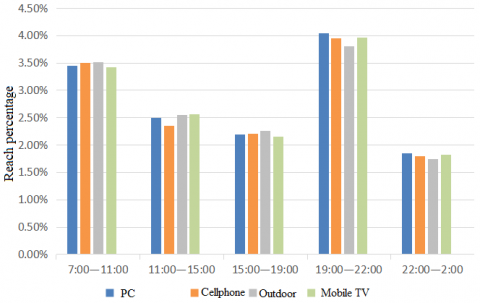Figure 5. Comparison of reach percentages at different advertising times

## 6.png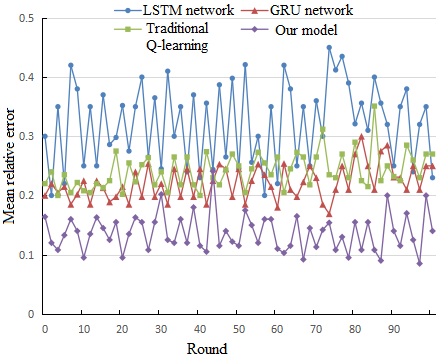Figure 6. Comparison of mean relative errors in different rounds

## 7.pngFigure 7. Comparison of mean relative errors in each round

## 8.png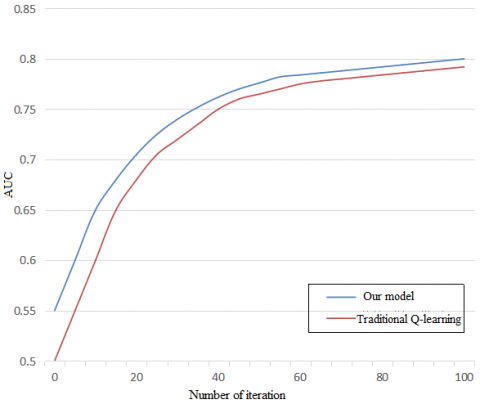Figure 8. The AUC trends of traditional Q-learning and our model

6. Conclusions

Based on Q-learning algorithm, this paper constructs a prediction model for advertising precision. Specifically, the erroneous, redundant, and missing items of the advertising data were preprocessed, the correlations between the data in the advertising data sequence were sorted out, and the process of feature construction and optimization was detailed. Experimental results show that, through feature construction and optimization, our model can make accurate predictions, whiling reducing data redundancy.

Next, the advertising precision prediction model was constructed in three steps based on Q-learning algorithm: setting up the state space and actions space, determining the state-action relationship with the DQN, and assigning weights to indices. Through repeated experiments, our model was found to achieve a lower mean relative error and a higher accuracy than the other methods.

References

 5G Automotive Association. (2019). C-V2X use cases methodology, examples and service level requirements. Whitepaper, Jun.

 Karlsson, N. (2016). Control problems in online advertising and beneﬁts of randomized bidding strategies. European Journal of Control, 30: 31-49. https://doi.org/10.1016/j.ejcon.2016.04.007

 Guo, J.X., Karlsson, N. (2017). Model reference adaptive control of advertising systems. In Proceedings of the 2017 American Control Conference, Seattle, WA, USA, ACC ’17, Seattle, WA, USA. https://doi.org/10.23919/ACC.2017.7963807

 Karlsson, N. (2018). Control of periodic systems in online advertising. In Proceedings of the 2018 IEEE 57th Conference on Decision and Control, Miami, FL, USA, CDC’18, Miami Beach, FL, USA. https://doi.org/10.1109/CDC.2018.8619536

 Karlsson, N. (2017). Plant gain estimation in online advertising processes. In Proceedings of the 2017 IEEE 56th Conference on Decision and Control, Melbourne, Australia, CDC’17, pp. 2182-2187. https://doi.org/10.1109/CDC.2017.8263968

 He, H., Karlsson, N. (2019). Identiﬁcation of seasonality in Internet trafﬁc to support control of online advertising. In Proceedings of the American Control Conference, Philadelphia, Philadelphia, USA, ACC. https://doi.org/10.23919/ACC.2019.8814710

 Quinn, P. (2017). Global Digital OOH Media Revenues Pacing Up 13% in 2017, US DOOH Advertising Expands 10%:PQ Media. Accessed: Sep. 6, 2017.

 Xia, C.L., Guha, S., Muthukrishnan, S. (2016). Targeting algorithms for online social advertising markets. Proceedings of the 2016 IEEE/ACM International Conference on Advances in Social Networks Analysis and Mining, IEEE Press, San Francisco, CA, USA. https://doi.org/10.1109/ASONAM.2016.7752279

 Lee, K.H., Kanzawa, Y., Derry, M., James, M.R. (2018). Multi-target track-to-track fusion based on permutation matrix track association. IEEE IV, Changshu, China. https://doi.org/10.1109/IVS.2018.8500433

 Scheel, A., Dietmayer, K. (2019). Tracking multiple vehicles using a variational radar model. IEEE Transactions on Intelligent Transportation Systems, 20(10): 3721-3736. https://doi.org/10.1109/TITS.2018.2879041

 Rangesh, A., Trivedi, M.M. (2019). No blind spots: Full-surround multi-object tracking for autonomous vehicles using cameras & Li DARs. IEEE Transactions on Intelligent Vehicles, 4(4): 588-599. https://doi.org/10.1109/TIV.2019.2938110

 Sutton, R., Barto, A. (2018). Reinforcement Learning: An Introduction. MIT Press, 342. https://doi.org/10.1007/978-1-4615-3618-5_1

 Redmon, J., Farhadi, A. (2018). YOLOv3: an incremental improvement. Computer Vision and Pattern Recognition.

 Hasselt, H.V., Guez, A., Silver, D. (2016). Deep reinforcement learning with double q-learning. AAAI Conference on Artificial Intelligence.

 Velickovic, P., Cucurull, G., Casanova, A., Romero, A., Lio, P., Bengio, Y. (2017). Graph attention networks. arXiv.org, Computer Science, Machine Learning.

 Berg, R.V.D., Kipf, T.N., Welling, M. (2017). Graph convolutional matrix completion. arXiv.org, Computer Science, Machine Learning.

 Lillicrap, T.P., Hunt, J.J., Pritzel, A., Heess, N., Erez, T., Tassa, Y., Silver, D., Wierstra, D. (2019). Continuous control with deep reinforcement learning. arXiv.org, Computer Science, Machine Learning.

 Cisco, F. (2009). Cisco Visual Networking Index Global Mobile Data Trafﬁc Forecast Update, 2017-2022White Paper. San Jose, CA.

 Matta, V., Mauro, M.D., Longo, M. (2017). DDoS Attacks with Randomized Trafﬁc Innovation: Botnet Identiﬁcation Challenges and Strategies. IEEE Transactions on Information Forensics and Security, 12(8): 1844-1859.

 Aseeri, A., Netjinda, N., Hewett, R. (2017). Alleviating Eavesdropping Attacks in Software-Deﬁned Networking Data Plane. CISRC '17: Proceedings of the 12th Annual Conference on Cyber and Information Security, pp. 1-8. https://doi.org/10.1145/3064814.3064832

 Duan, Q., Al-Shaer, E., Chatterjee, S., Halappanavar, M., Oehmen, C.S. (2018). Proactive routing mutation against stealthy Distributed Denial of Service attacks: metrics, modeling, and analysis. The Journal of Defense Modeling and Simulation, 15(2): 219-230. https://doi.org/10.1177/1548512917731002

 Mao, H.Z., Netravali, R., Alizadeh, M. (2017). Neural adaptive video streaming with Pensieve. Neural Adaptive Video Streaming with Pensieve, 2017: 197-210. https://doi.org/10.1145/3098822.3098843

 Xiao, Y., Krunz, M., Volos, H., Bando, T. (2019). Driving in the fog: Latency measurement, modeling, and optimization of LTE-based fog computing for smart vehicles. IEEE SECON, Boston, MA, USA. https://doi.org/10.1109/SAHCN.2019.8824922

 Lee, G., Saad, W., Bennis, M. (2019). An online optimization framework for distributed fog network formation with minimal latency. IEEE Transactions on Wireless Communications, 18(4): 2244-2258. https://doi.org/10.1109/TWC.2019.2901850

 Zeng, T.C., Semiari, O., Saad, W., Bennis, M. (2019). Joint communication and control for wireless autonomous vehicular platoon systems. IEEE Transactions on Communications Early Access, 67(11): 7907-7922. https://doi.org/10.1109/TCOMM.2019.2931583